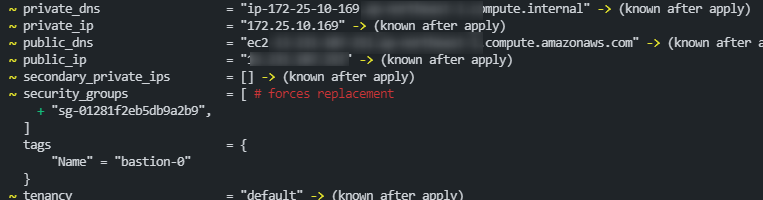# 結論

VPCEC2インスタンス作成する定義の場合は、`security_groups`でなく`vpc_security_group_ids`でセキュリティグループを指定しましょう。

# 事象

お題は以下のコード

## コード

```resource "aws_instance" "bastion" {
count = var.host_count

ami                         = data.aws_ssm_parameter.amzn2_ami.value
instance_type               = "t3.nano"
key_name                    = aws_key_pair.my_key.id
subnet_id                   = aws_subnet.prac_public.id
security_groups             = [aws_security_group.allow_ssh_icmp.id]

tags = {
Name = "bastion-\${count.index}"
}
}```

なんなら、定義ファイルを一切変更しなくても、`terraform plan`すると、"no changes"とならずに以下のように「replaced」となってしまう。

## 動作

```\$ terraform plan

[...]

Terraform will perform the following actions:

# aws_instance.bastion must be replaced
-/+ resource "aws_instance" "bastion" {
~ arn                          = "arn:aws:ec2:***:****:instance/i-****" -> (known after apply)
~ availability_zone            = "****" -> (known after apply)
~ cpu_core_count               = 1 -> (known after apply)
~ cpu_threads_per_core         = 2 -> (known after apply)
- disable_api_termination      = false -> null
- ebs_optimized                = false -> null
- hibernation                  = false -> null
+ host_id                      = (known after apply)
- iam_instance_profile         = "" -> null
~ id                           = "i-********" -> (known after apply)
~ instance_state               = "running" -> (known after apply)
~ ipv6_address_count           = 0 -> (known after apply)
~ ipv6_addresses               = [] -> (known after apply)
- monitoring                   = false -> null
~ outpost_arn                  = "" -> (known after apply)
~ password_data                = "" -> (known after apply)
~ placement_group              = "" -> (known after apply)
~ primary_network_interface_id = "eni-****" -> (known after apply)
~ private_dns                  = "ip-172-25-10-169.***.compute.internal" -> (known after apply)
~ private_ip                   = "172.25.10.169" -> (known after apply)
~ public_dns                   = "ec2-********.compute.amazonaws.com" -> (known after apply)
~ public_ip                    = "********" -> (known after apply)
~ secondary_private_ips        = [] -> (known after apply)
~ security_groups              = [ # forces replacement
+ "sg-01281f2eb5db9a2b9",
]
tags                         = {
"Name" = "bastion-0"
}
~ tenancy                      = "default" -> (known after apply)
~ vpc_security_group_ids       = [
- "sg-01281f2eb5db9a2b9",
] -> (known after apply)
# (7 unchanged attributes hidden)

- credit_specification {
- cpu_credits = "unlimited" -> null
}

+ ebs_block_device {
+ delete_on_termination = (known after apply)
+ device_name           = (known after apply)
+ encrypted             = (known after apply)
+ iops                  = (known after apply)
+ kms_key_id            = (known after apply)
+ snapshot_id           = (known after apply)
+ tags                  = (known after apply)
+ throughput            = (known after apply)
+ volume_id             = (known after apply)
+ volume_size           = (known after apply)
+ volume_type           = (known after apply)
}

~ enclave_options {
~ enabled = false -> (known after apply)
}

+ ephemeral_block_device {
+ device_name  = (known after apply)
+ no_device    = (known after apply)
+ virtual_name = (known after apply)
}

~ http_endpoint               = "enabled" -> (known after apply)
~ http_put_response_hop_limit = 1 -> (known after apply)
~ http_tokens                 = "optional" -> (known after apply)
}

+ network_interface {
+ delete_on_termination = (known after apply)
+ device_index          = (known after apply)
+ network_interface_id  = (known after apply)
}

~ root_block_device {
~ delete_on_termination = true -> (known after apply)
~ device_name           = "/dev/xvda" -> (known after apply)
~ encrypted             = false -> (known after apply)
~ iops                  = 100 -> (known after apply)
+ kms_key_id            = (known after apply)
~ tags                  = {} -> (known after apply)
~ throughput            = 0 -> (known after apply)
~ volume_id             = "vol-0ca9e88e004237231" -> (known after apply)
~ volume_size           = 8 -> (known after apply)
~ volume_type           = "gp2" -> (known after apply)
}
}

Plan: 1 to add, 0 to change, 1 to destroy.```## 対応

aws_instanceリソースのsecurity_groupsオプションの項のドキュメントを確認すると以下の通り、`vpc_security_group_ids`を使うように書いてある。

NOTE:

If you are creating Instances in a VPC, use `vpc_security_group_ids` instead.

ということでコード変更。

```--- a/aws/practice/ec2.tf
+++ b/aws/practice/ec2.tf
@@ -14,7 +14,7 @@ resource "aws_instance" "bastion" {
instance_type               = "t3.nano"
key_name                    = aws_key_pair.my_key.id
subnet_id                   = aws_subnet.prac_public.id
-  security_groups             = [aws_security_group.allow_ssh_icmp.id]
+  vpc_security_group_ids      = [aws_security_group.allow_ssh_icmp.id]

tags = {
```

この内容で`terraform plan`を実行すると、期待通り"no changes"になった。

```\$ terraform plan
aws_key_pair.my_key: Refreshing state... [id=***]
aws_vpc.practice: Refreshing state... [id=***]
aws_internet_gateway.gw: Refreshing state... [id=***]
aws_security_group.allow_ssh_icmp: Refreshing state... [id=***]
aws_subnet.prac_priv2: Refreshing state... [id=***]
aws_subnet.prac_public: Refreshing state... [id=***]
aws_subnet.prac_priv1: Refreshing state... [id=***]
aws_route_table.public_route: Refreshing state... [id=***]
aws_security_group_rule.egress: Refreshing state... [id=***]
aws_security_group_rule.ssh: Refreshing state... [id=***]
aws_security_group_rule.icmp: Refreshing state... [id=***]
aws_instance.bastion: Refreshing state... [id=***]
aws_route_table_association.public_subnet: Refreshing state... [id=***]

No changes. Infrastructure is up-to-date.```

これで作成済みEC2に影響しないコード変更を行ったときに、余計なEC2再作成が発生しなくなった。